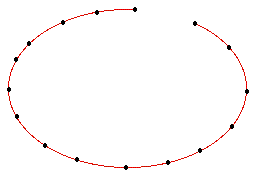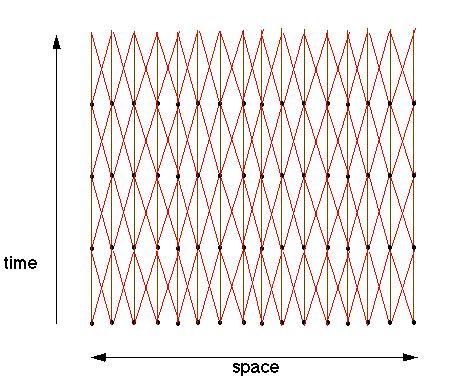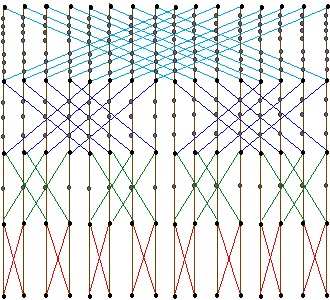## Parallel Execution of Recurrences: Linear Array

This shows how efficient prototyping of recurrences also can utilise the processing power of parallel machines.

### Static View of Linear Array

A linear array is a computer where the processing elements are connected in a linear fashion, i.e., each node is connected to two other nodes, except the two end nodes which are connected to just one neighbour. The figure shows a 16 node linear array connected computer### Space-Time View of Linear Array

If we try to imagine how the communications of a linear array may appear if we draw them schematically in time, we may get the following figure.Here all the processors of the linear array are depicted at every time-step, as if all computations where taking place instantaneously and synchronously. The communications now go between the timesteps, to the neighbours as defined by the static configuration of the linear array. These lines are marked with red, diagonal lines forward in time. In addition, a processor may retain information between computation steps. This is depicted as a communication from a processor to itself (brown vertical lines).

### Heatflow Problem Embedded in Linear Array

The one-dimensional heatflow computation may easily be embedded in the linear arrays computation structure. The dependencies of this computation exhibits the same graph as the communications of the linear array. An animated demo of this has been prepared as Java code.

### Butterfly Embedded in Linear Array

Butterfly computations can also be embedded in the linear array. This requires us to use more than one time-step for communications, and progressively more timesteps as the communications of the butterfly grow longer. This can be seen in the figure below, which shows a height 4 butterfly embedded in a 16 node linear array using 16 timesteps. The scale is logarithmic in the time-direction, and the processors have been shaded in grey when they do not compute but just forward communications.### The parallelisation technique

The embedding of a computation in a parallel machine over time
• maps computations to processors at specific time-steps,
• maps dependencies to paths in the parallel machine's space-time communication structure.
The mapping is user definable and expressible as program code. This gives the programmer full control over the computation, but still avoids lots of detail in the writing of parallel programs.

This shows some of the potential efficient prototyping of recurrences has for defining parallel programs.
© 1997 Magne Haveraaen, University of Bergen, Norway.